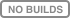# mlinsights: tricky scikit-learn#mlinsights implements functions to get insights on machine learned models or various kind of transforms to help manipulating data in a single pipeline. It implements `QuantileLinearRegression` which trains a linear regression with L1 norm non-linear correlation based on decision trees, `QuantileMLPRegressor` which is a modification of scikit-learn’s MLPRegressor which trains a multi-layer perceptron with L1 norm…

Short example:

<<<

```from sklearn.datasets import load_diabetes
from sklearn.linear_model import LinearRegression
from mlinsights.mlmodel import QuantileLinearRegression

X, y = data.data, data.target

clq = QuantileLinearRegression()
clq.fit(X, y)
print(clq.coef_)

clr = LinearRegression()
clr.fit(X, y)
print(clr.coef_)
```

>>>

```    [   5.75895899 -301.11702117  464.04300247  385.35409612 -805.26720203
425.19307405  110.3164599   245.8509531   738.51804393   35.6856007 ]
[ -10.0098663  -239.81564367  519.84592005  324.3846455  -792.17563855
476.73902101  101.04326794  177.06323767  751.27369956   67.62669218]
```

This documentation was generated with scikit-learn version…

<<<

```from sklearn import __version__
print(__version__)
```

>>>

```    1.1.1
```